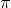The surface area of a soccer ball is 254.5 in?. What is the radius to the tenths’ place?

Question

The surface area of a soccer ball is 254.5 in?.
What is the radius to the tenths’ place?

in progress 0
5 months 2021-08-10T20:50:05+00:00 1 Answers 12 views 0

4.5 inches

Step-by-step explanation:

Use the formula for the surface area of a sphere, SA = 4Plug in the surface area and solve for r, the radius:

SA = 4254.5 =20.25 = r²

4.5 = r

So, the radius is approximately 4.5 inches